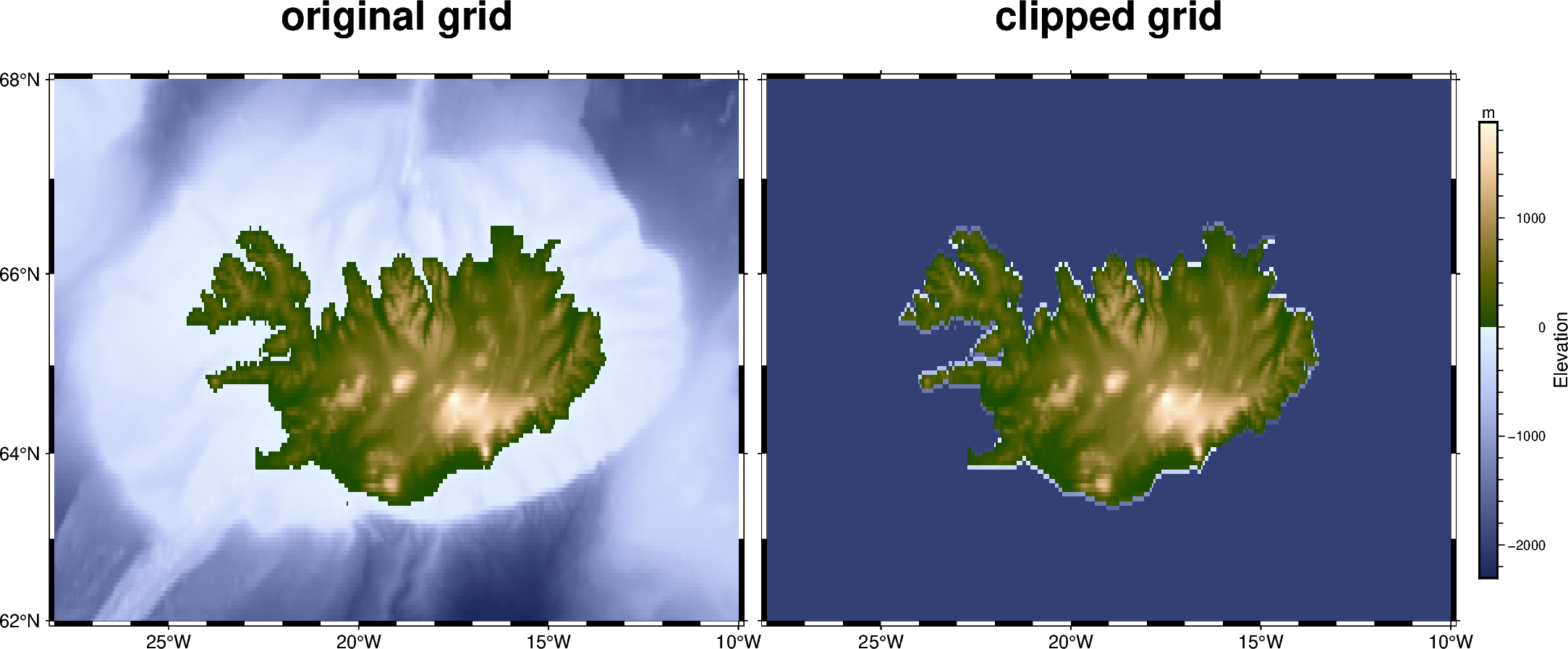# Clipping grid values

The `pygmt.grdclip` method allows to clip defined ranges of grid values. In the example shown below we set all elevation values (grid points) smaller than 0 m (in general the bathymetric part of the grid) to a common value of -2000 m via the `below` parameter.Out:

```<IPython.core.display.Image object>
```

```import pygmt

fig = pygmt.Figure()

# Define region of interest around Iceland
region = [-28, -10, 62, 68]

# Load sample grid (3 arc minute global relief) in target area

# Plot original grid
fig.basemap(region=region, projection="M12c", frame=["f", '+t"original grid"'])
fig.grdimage(grid=grid, cmap="oleron")

# Shift plot origin of the second map by "width of the first map + 0.5 cm"
# in x direction
fig.shift_origin(xshift="w+0.5c")

# Set all grid points < 0 m to a value of -2000 m.
grid = pygmt.grdclip(grid, below=[0, -2000])

# Plot clipped grid
fig.basemap(region=region, projection="M12c", frame=["f", '+t"clipped grid"'])
fig.grdimage(grid=grid)
fig.colorbar(frame=["x+lElevation", "y+lm"], position="JMR+o0.5c/0c+w8c")

fig.show()
```

Total running time of the script: ( 0 minutes 2.377 seconds)

Gallery generated by Sphinx-Gallery